matlab教程 订阅
《MATLAB教程R2012a》是2010年北京航空航天大学出版社出版的图书，作者是张志涌、杨祖樱。 展开全文《MATLAB教程R2012a》是2010年北京航空航天大学出版社出版的图书，作者是张志涌、杨祖樱。

355

43.00

MATLAB教程R2012a

2010-08

16开

ISBN
978-7-5124-0145-7
MATLAB教程R2012a内容简介

• matlab基础教程，适合入门级别，主要讲了matlab矩阵的基本操作，数值计算与符号运算，内核引擎调用等
• 该文档为matlab入门教程，讲述的内容简单易懂，有几本的语法介绍和高级的使用讲解，对于初学者有一定的帮助作用
• MATLAB学习PDF，适用于MATLAB 7.1版本,可以用来较好入门
• 台大的郭彦甫教授上课的ppt教程，对于matlab学习有很大帮助，视频在bili,很好的课程。
• 第一章 Matlab中的数组操作，第二章 matlab程序设计，第三章 matlab图形功能，第四章 拟合与插值，第五章 图与网络分析模型选讲，第六章 积分与数值积分，第七章 方程与微分方程，第八章 matlab在概率统计中的应用；...
• matlab教程ppt(全) matlab教程ppt(全)matlab教程ppt(全)matlab教程ppt(全)
• MATLAB 语言程序设计基础，MATLAB 语言是当前国际上自动控制领域的首选 计算机语言，也是很多理工科专业最适合的计算机数 学语言。
• MATLAB信号处理详解 结合MATLAB最新版本系统地介绍信号处理及现代信号处理或者非平稳信号处理(包括信号处理、阵列信号处理、时频分析及高阶谱分析)的基本理论及在工程应用中的一些基本方法；详细地介绍MATlLAB工具箱...
• 适用于《MATLAB教程及实训（第3版）》曹弋，对《MATLAB教程及实训（第2版）》曹弋也是通用的（电子课件+模型文件）
• MATLAB教程及实训（第2版）》曹弋的课后习题答案，对于《MATLAB教程及实训（第3版）》曹弋也是适用的。
• matlab入门教程，pdf版，内以幻灯片模式呈现。图形图像处理及其实现
• SPIE出版，用于解决计算傅立叶光学问题，理论和Matlab案例相结合，内容扎实详细。英文版，但是帮助很大。
• matlab教程张志勇,关于matlab使用的教程，免费下载。希望有人需要它
• ## MATLAB教程（1） MATLAB 基础知识

万次阅读 多人点赞 2017-10-26 20:57:32
为了加深自己的印象，扎实基础，现将官网上的基础教程做简单的翻译。 首先，以下从九个部分简单介绍基础入门知识。第一部分：MATLAB显示桌面的基本布局...

初学。去年看过一点点MATLAB，很久不用，遗忘惊人。为了加深自己的印象，扎实基础，现将官网上的基础教程做简单的翻译。

首先，以下从九个部分简单介绍基础入门知识。
第一部分：MATLAB显示桌面的基本布局
https://cn.mathworks.com/help/matlab/learn_matlab/desktop.html
1.启动MATLAB后，桌面会显示默认布局，主要有以下三个部分：
当前文件夹命令行窗口工作空间
2.在MATLAB中一般需要创建变量和调用函数。
直接在命令行中输入变量名以及其值即可（比如：a = 1）,多个变量的创建同理，不再赘述。
需要注意的是，如果没有定义输出变量，MATLAB会使用一个名为ans的变量来储存计算结果。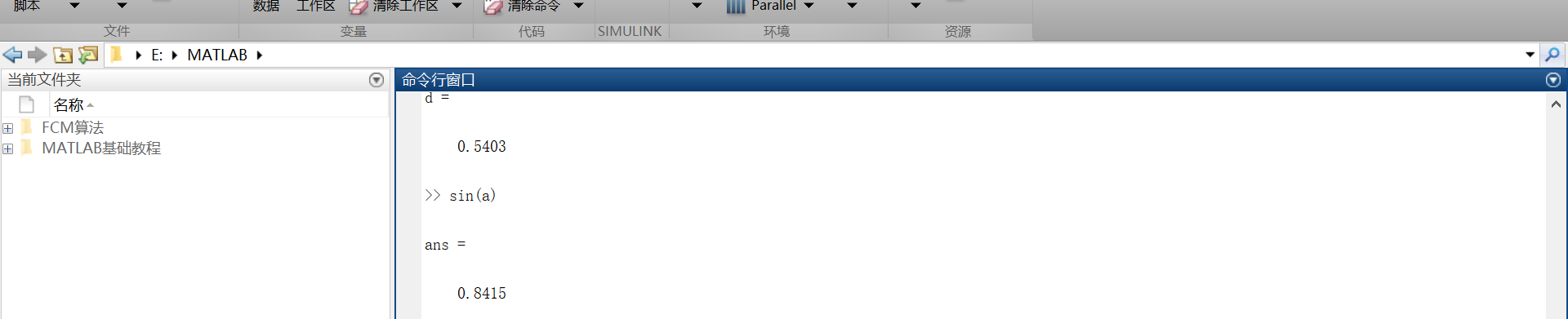3.如果语句以分号结束，表示MATLAB内部会执行这一运算，但是命令行不对计算结果进行输出。
第二部分：矩阵和数组
https://cn.mathworks.com/help/matlab/learn_matlab/matrices-and-arrays.html
大多数的编程语言一次性只能对一个数进行操作，而MATLAB主要被用来对整个矩阵或者数组进行操作。不管是什么类型的数据，所有的额MATLAB变量都是数组。矩阵是常用于线性代数的二维数组。
1.数组的创建
在一个单行中创建一个拥有四个元素的数组，其各个元素之间用逗号或者空格隔开。  例如：  a = [1 2 3 4]  这种数组叫做单行矩阵（行向量）。创建一个多行矩阵，其各个行之间用分号隔开。  例如：  b = [1,2;3,4;5,6]还可以用函数来创建矩阵。  例如：创建一个5行1列每一个元素都是0的列向量。  z = zero(5,1)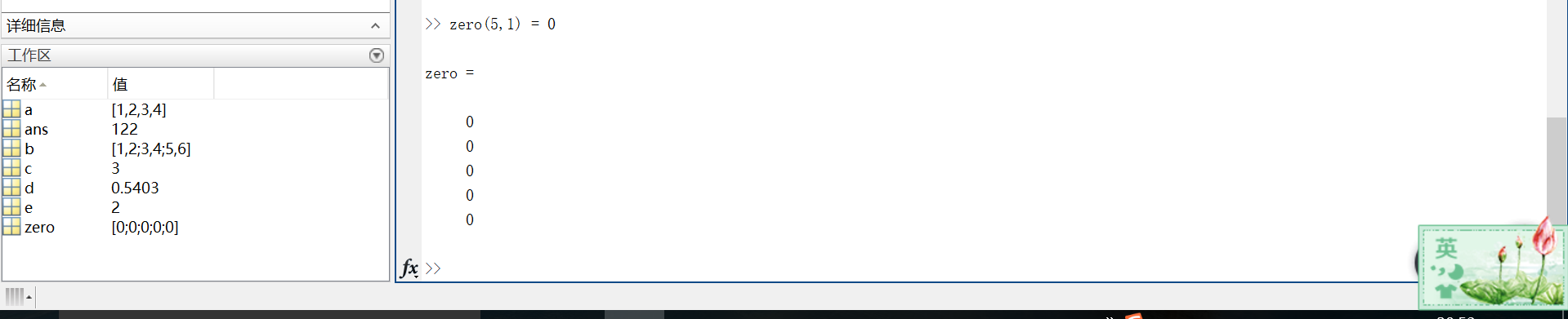2.矩阵和数组的操作
在MATLAB中，允许用一个单一的运算或者函数对矩阵中所有的数据进行处理（操作）。
例如：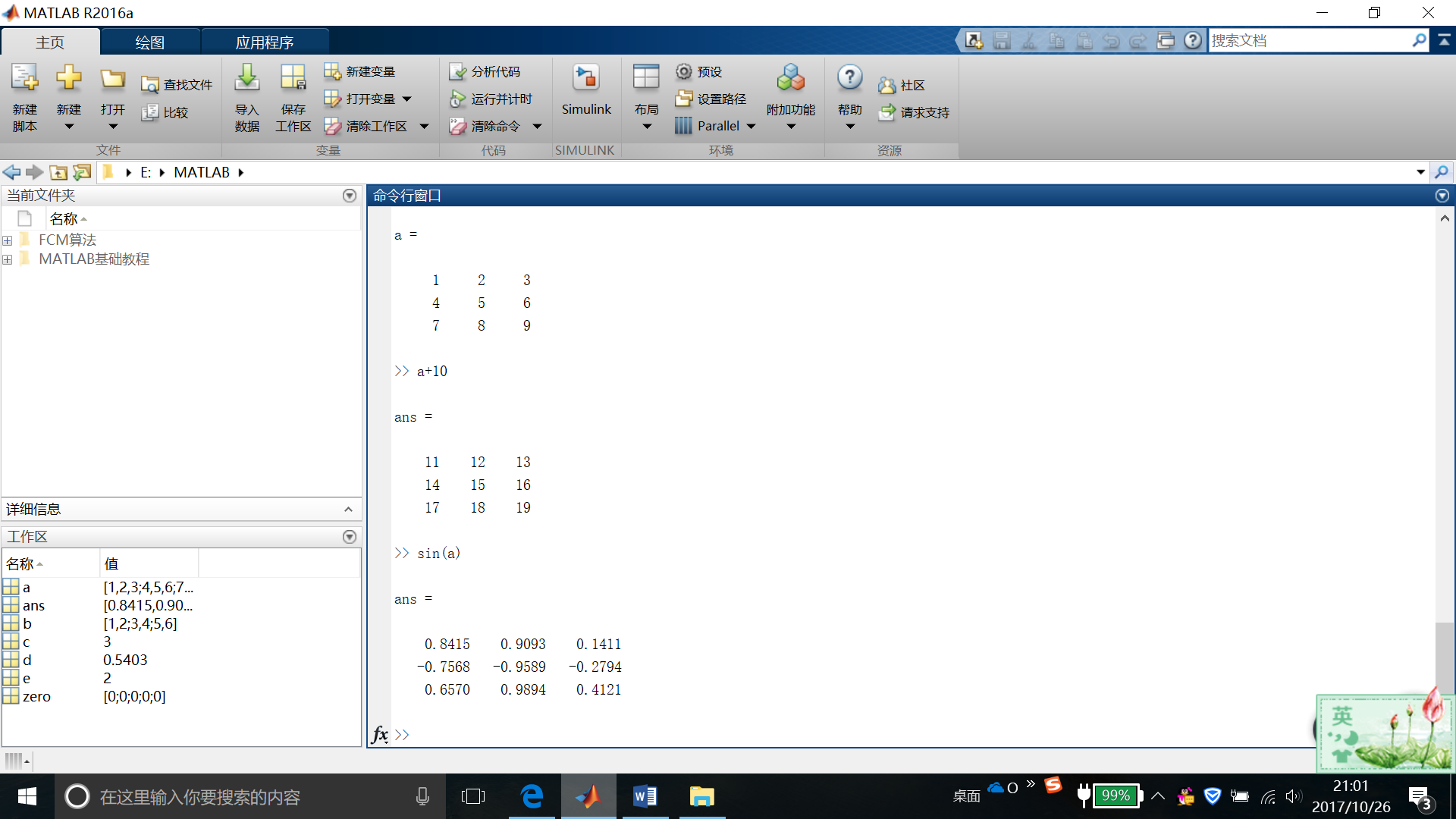如果要对矩阵进行转置，则使用单引号(‘)  例如：a’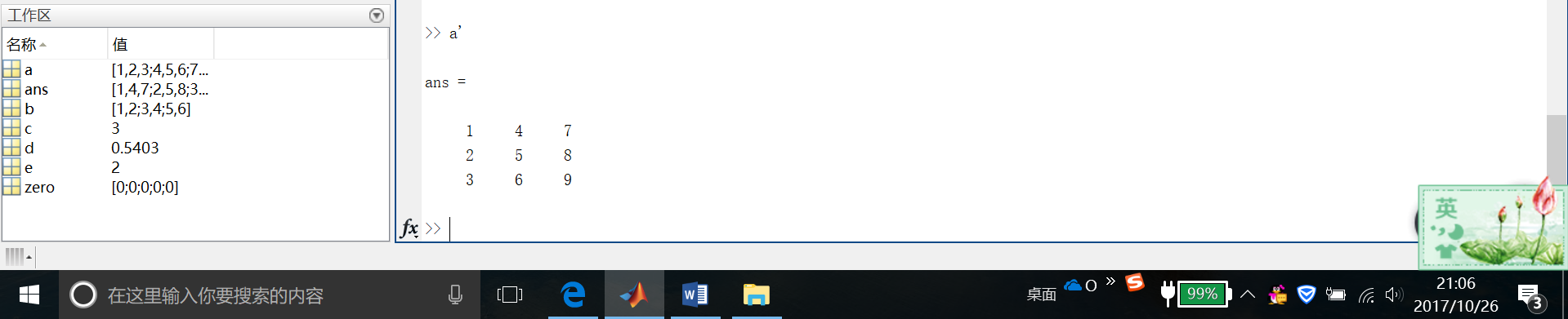可以通过执行标准矩阵乘法，通过*运算符来计算内积。
这是我自己的结果截图……很明显是不对滴~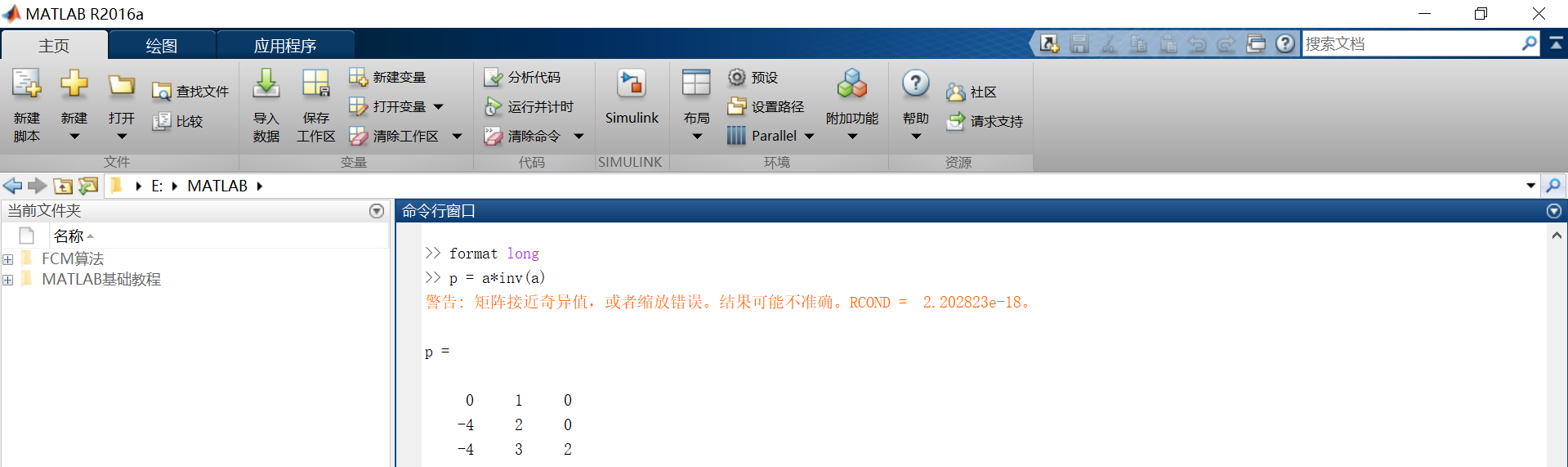然后下面是官网爸爸给的运行结果。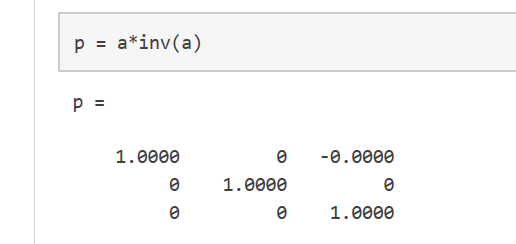我们都知道，一个矩阵与它的逆矩阵相乘的结果的确应该等于单位矩阵。至于这里为什么不对，还希望各位大佬们能不吝赐教，大恩不言谢~
这里要注意的是，p不是一个整数值矩阵，MATLAB存储数字是以浮点型存储的，真实值和它的浮点数在运算时有小小的不同，我们可以使用格式化命令，显示更多的小数位数。

比如：  format long  p = a*inv(a)

可以使用format short来重置到较短格式的使用。
x = a.*a用来表示对矩阵的每一个元素做平方操作。  若需要对每一个元素做立方运算，则用x = a.^3(如图)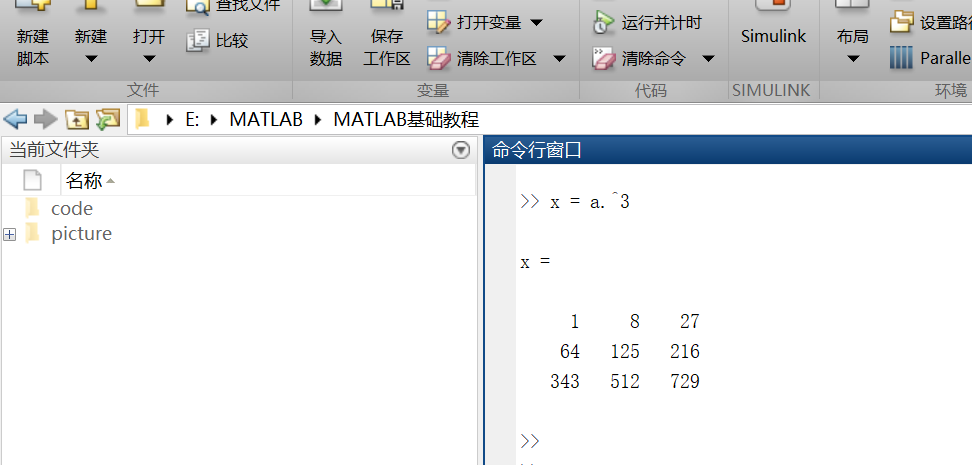矩阵的扩充  行方向上：A = [a,a]  列方向上：A = [a; a]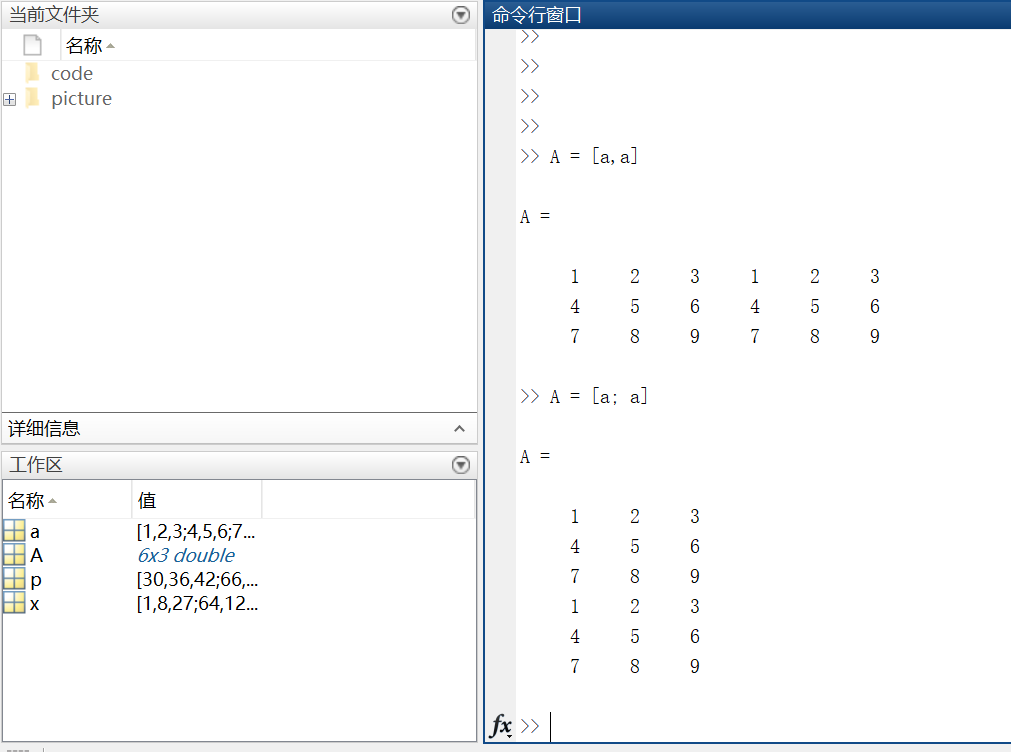复数  复数由实部和虚部组成，它的虚部是-1的平方根。  即sqrt(-1) = 0.0000 + 1.0000i  一般用i或者j来代表复数的虚部部分。
第三部分：数组的索引
https://cn.mathworks.com/help/matlab/learn_matlab/array-indexing.html
MATLAB中的每一个变量都是可以存储多个值的数组。如果想要使用数组中的某一个值，则需要用到索引。
1.单个元素的索引
从一个数组中索引某个元素一般有两种方法：  第一种比较常见：数组名加要索引的元素所在的行和列，例如A(2，4) = 8。  第二种不太常用：数组名加一个下标，该下标按列遍历。A(14) = 8。【这里不太好表达，上图吧~】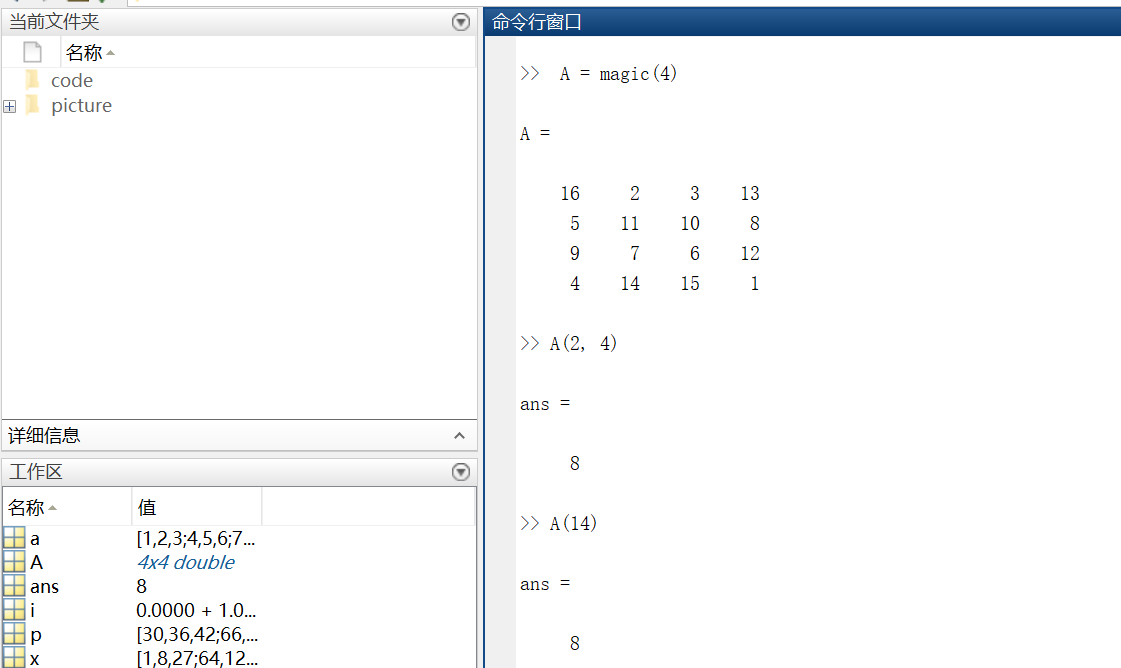再多说一句，其实A(14)中的14表示按列数第14个元素！  利用下标索引元素这种方式叫做线性索引。  注意：如果索引超出矩阵维度，MATLAB会报错。
2.多个元素的索引  引用数组的多个元素，使用冒号操作符m : n(m，n分别表示索引开始和结束的范围)
例1索引第二列第1个元素到第3个元素。  例2索引第三行全部元素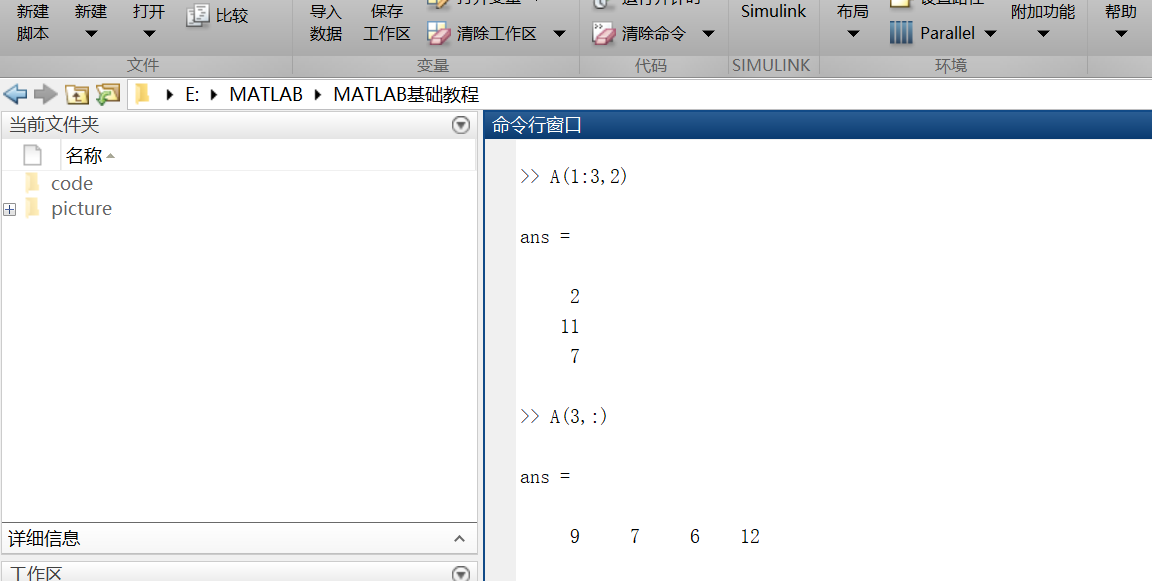不难发现，索引行则将行数放在逗号前；索引列要将列数放在逗号后。
另外，冒号运算符也可以帮助创建一组等距的矢量值，例如：  B = 0:10:100  —->B = 0 10 20 30 40 50 60 70 80 90 100  输出一个从0到100步长为10的数组。
第四部分：工作空间变量
https://cn.mathworks.com/help/matlab/learn_matlab/workspace.html
工作区包含你创建的变量或者从数据文件或其他程序导入到MATLAB中的变量。例如，我现在创建两个变量 ：  A = magic(4)  B = rand(3,5,2)
我们可以通过whos来看工作空间的内容。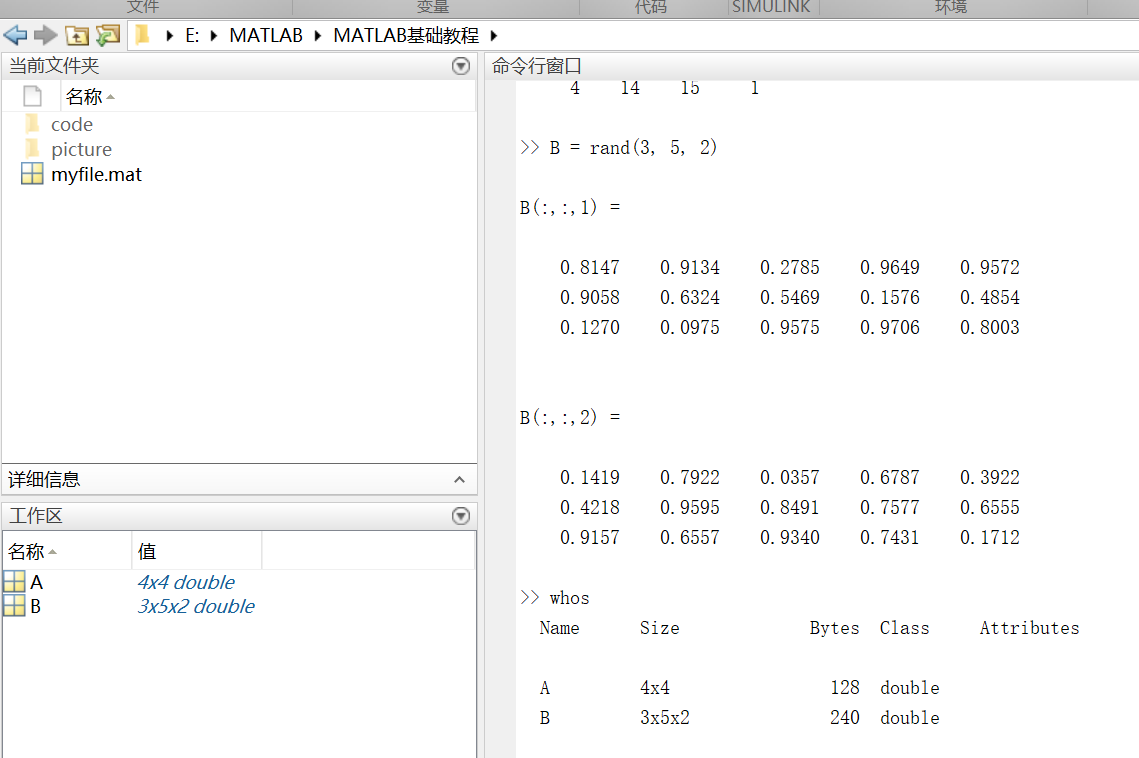第五部分：文本和字符
https://cn.mathworks.com/help/matlab/learn_matlab/character-strings.html
在处理文本时，将其中的字符序列用单引号括起来，可以将文本分配给变量。  例如：myText = ‘Hello, world’;如果文本本身包括一个单引号，则在定义时使用两个单引号。  例如：otherText = ‘You”re right’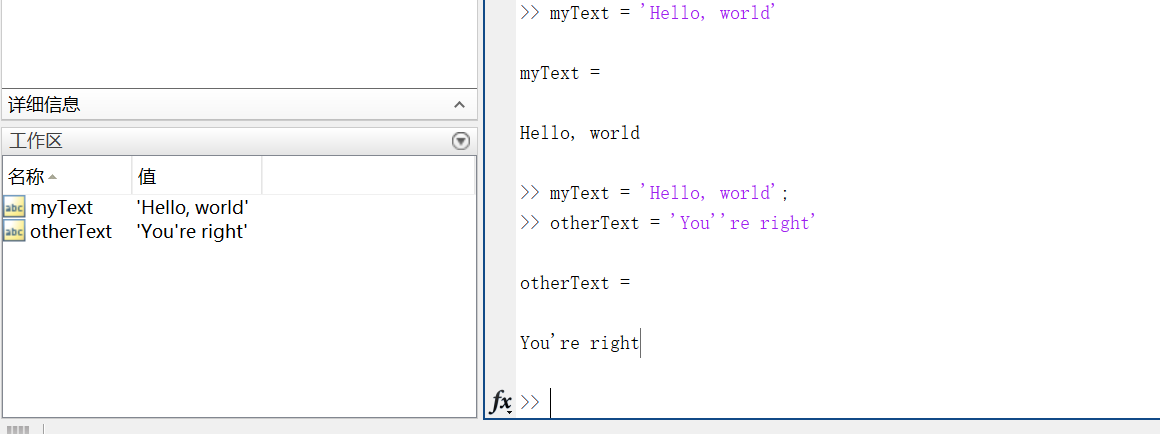同所有的MATLAB变量一样，上述创建的两个变量也都是数组，他们的数据类型是字符型。注意：字符数组也可以像数字数组那样用方括号括起来表示。
要将数字转换成字符，就要用到函数。例如num2str()或者int2str()。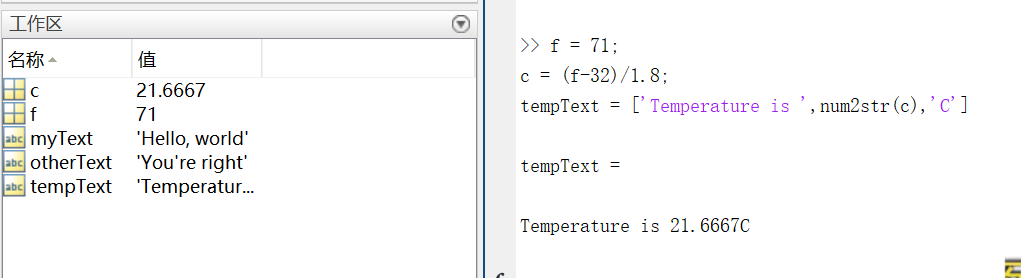第六部分：函数调用
https://cn.mathworks.com/help/matlab/learn_matlab/calling-functions.html
MATLAB提供了大量函数来执行计算任务，函数相当于其他编程语言中的子程序或方法。  例如求最大值的函数，max()。
将输入参数用括号括起来，如果有多个参数，则将参数用逗号隔开。  一般通过将函数值赋给某个变量这种方式来返回（输出）函数值，如果有多个输出参数，则需将它们用方括号括起来。  例如：  A = [1 3 5];  [maxA,location] = max(A)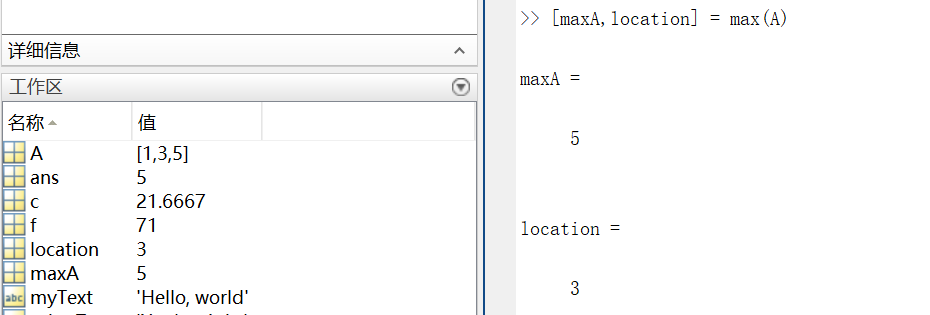可以用单引号括住任何字符进行输入。  例如：  disp(‘hello world’)  ===>输出 hello world

disp()用来显示变量的内容,括号里可以是字符,结构体,矩阵等等，类似于c语言的printf()。

clc函数被用来清空命令行。
第七部分：二、三维图
https://cn.mathworks.com/help/matlab/learn_matlab/plots.html
折线图 （1） 画图 x = 0:pi/1000:2*pi;  y = sin(x);  plot(x,y)
这里x就用到了前面说到的索引。x表示0到2*pi之间步长为pi/100的值。  二维图如下：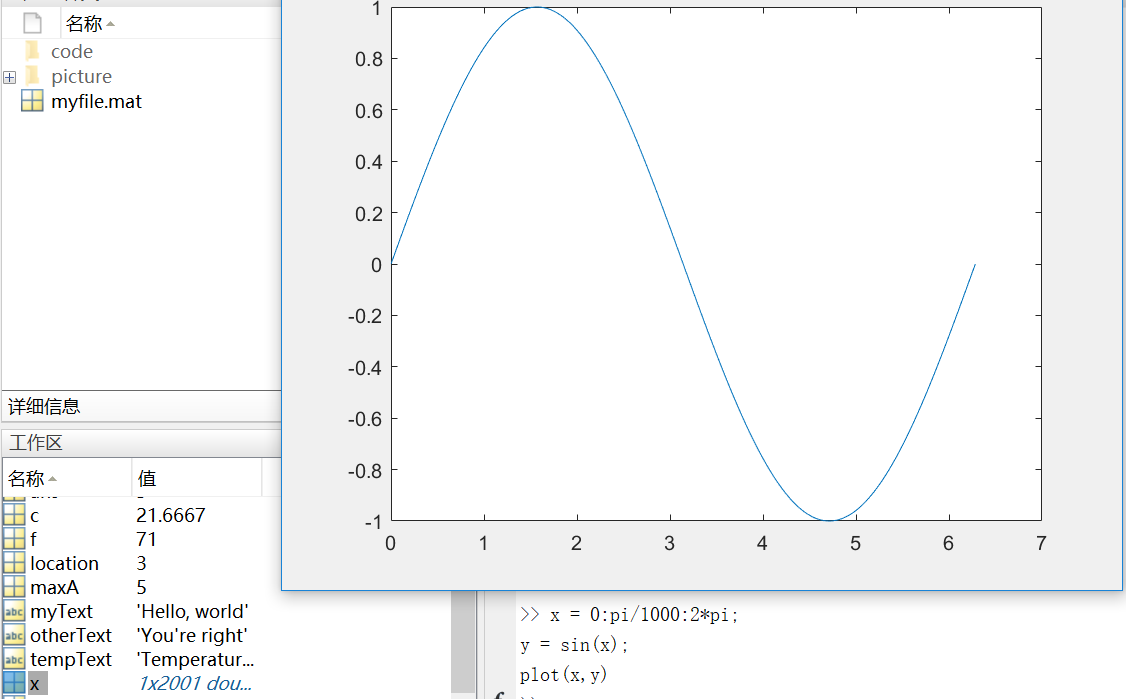这里多说一下步长，步长决定了横轴取值的密集程度。下图是将步长改为pi/10之后的图像。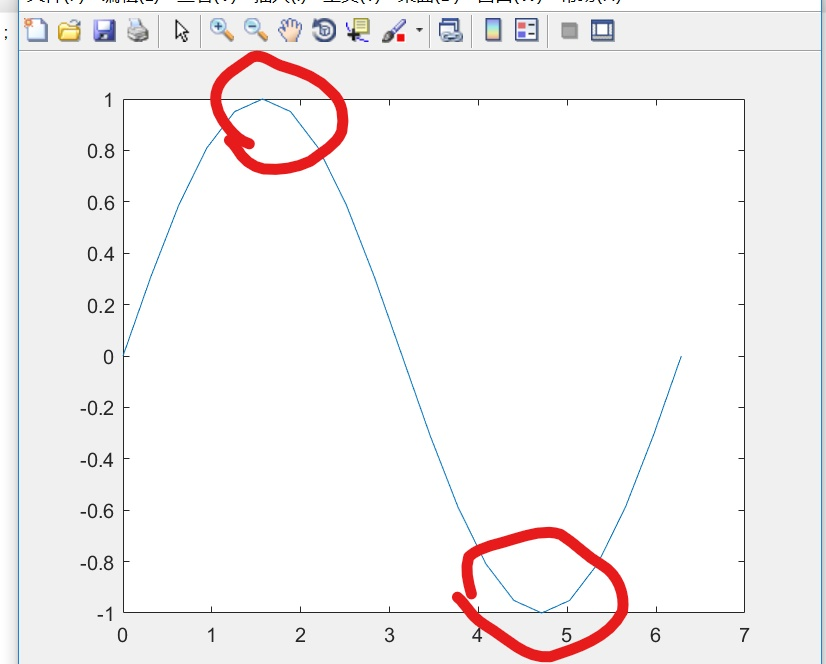对比图中标记处不难发现，步长增大，图像的精确度变低，所以这里尽量保证步长足够小，使得x可以取到足够多的值
（2） 坐标  我们可以对横轴和纵轴分别命名，并对上述正弦图像加上标题。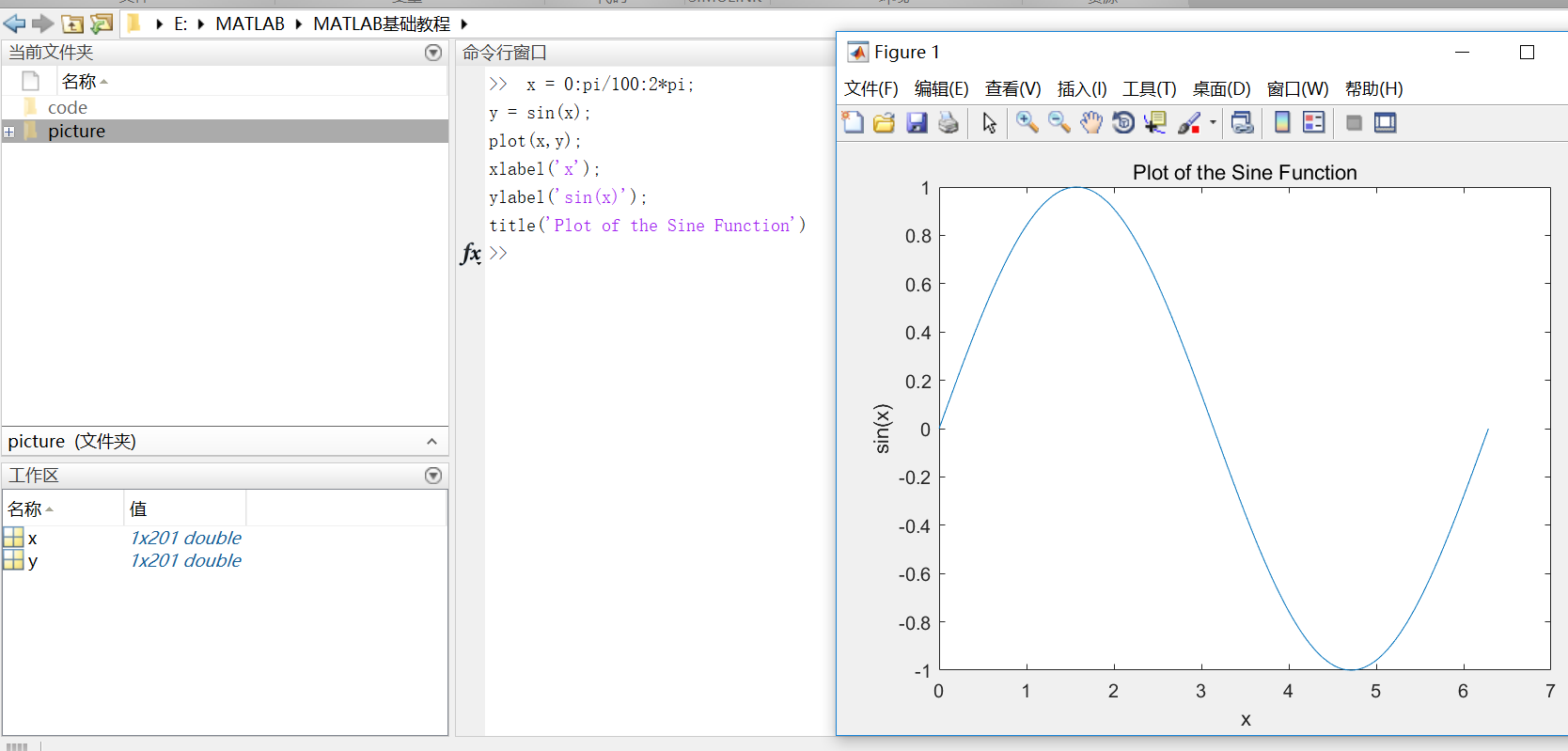（3）plot(x, y, ‘r–’)  通过在图像函数中添加第三个输入参数，可以用红色虚线绘制与原来相同的变量。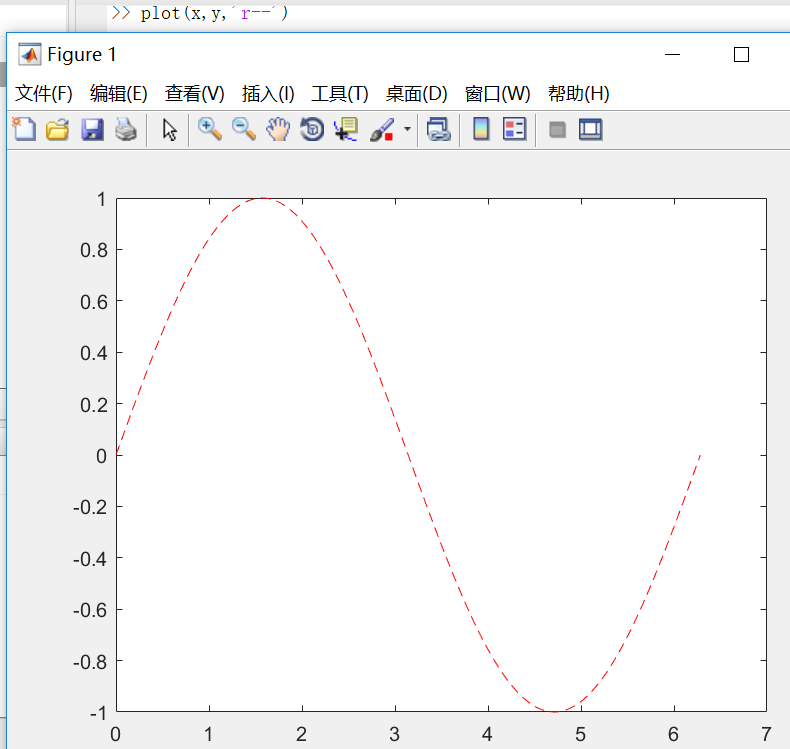其中第三个参数规定了线条的颜色、样式和标记的字符。（例如上例中的‘r–’表示红色虚线。）标记是指对每一个数据点用参数所给的标记形式进行表示。（例如’g:o’表示用‘o’连成一条绿色的线输出=.=我这个解释没谁了，讲不清楚还是上图吧…..）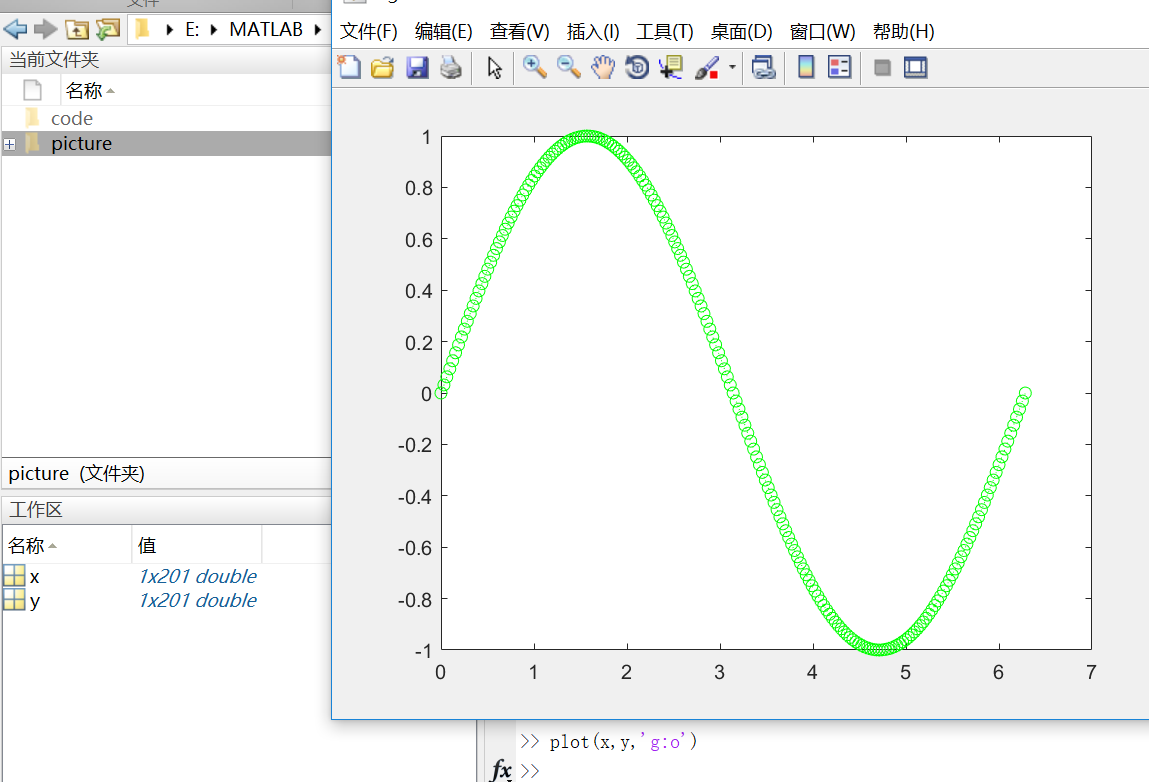这样的标记除了’o’，还有‘+’，‘*’等等。
这时候你会发现，我们开始为第一个图定义的标题和标签不再显示在当前图形窗口中了。这是因为在默认情况下，重新调用一个绘图函数，MATLAB就会清除数据，重置轴和其他元素来准备新的绘图。  若要将图添加到现有图形，使用hold命令即可。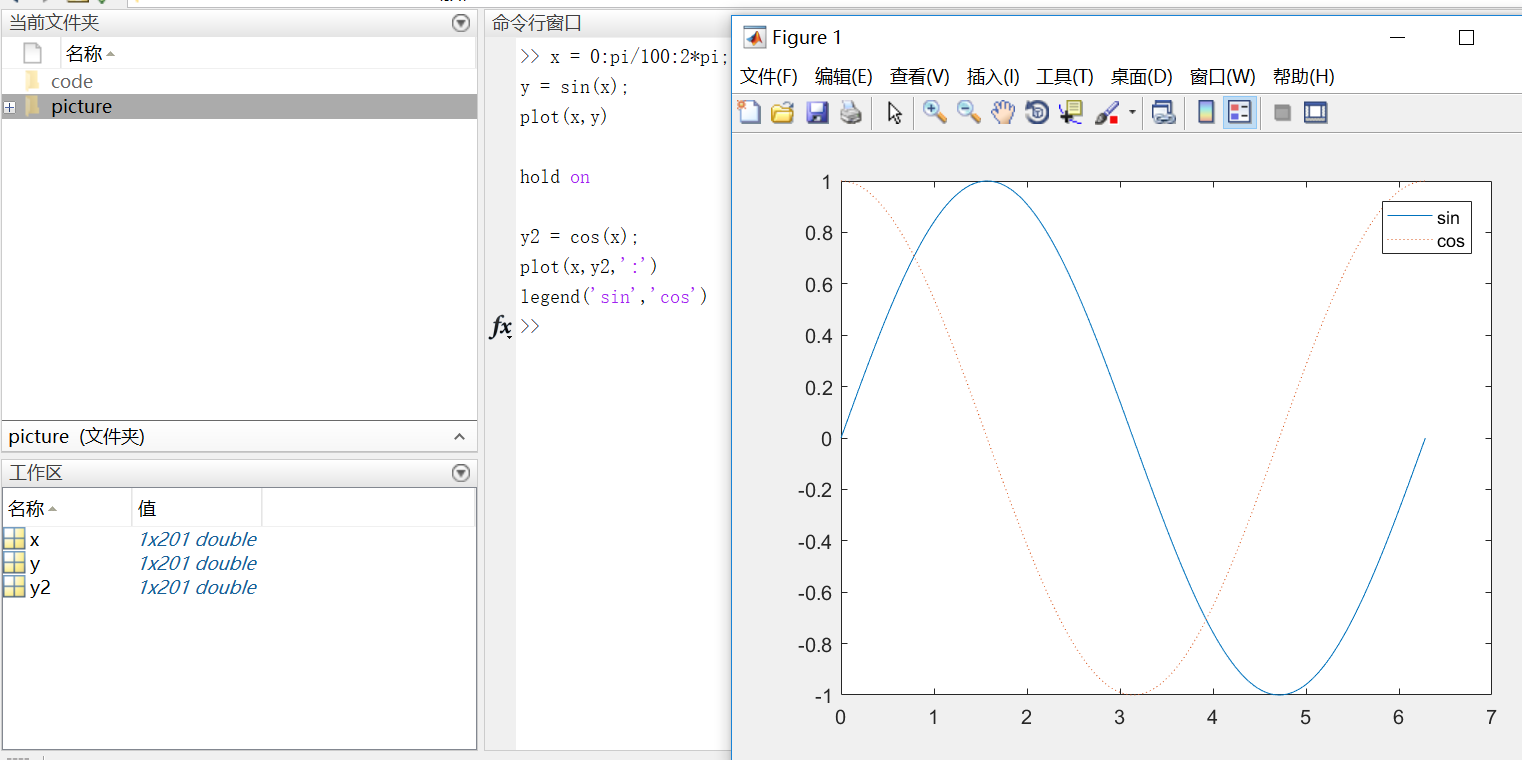在使用hold off或close the window命令之前，所有的图都会出现在当前的图形窗口中。
3D图像 3D图像通常用有两个参数的函数来定义一个曲面，z = f(x,y) 。  对于z，首先使用meshgrid命令在函数定义域内创建若干(x,y)。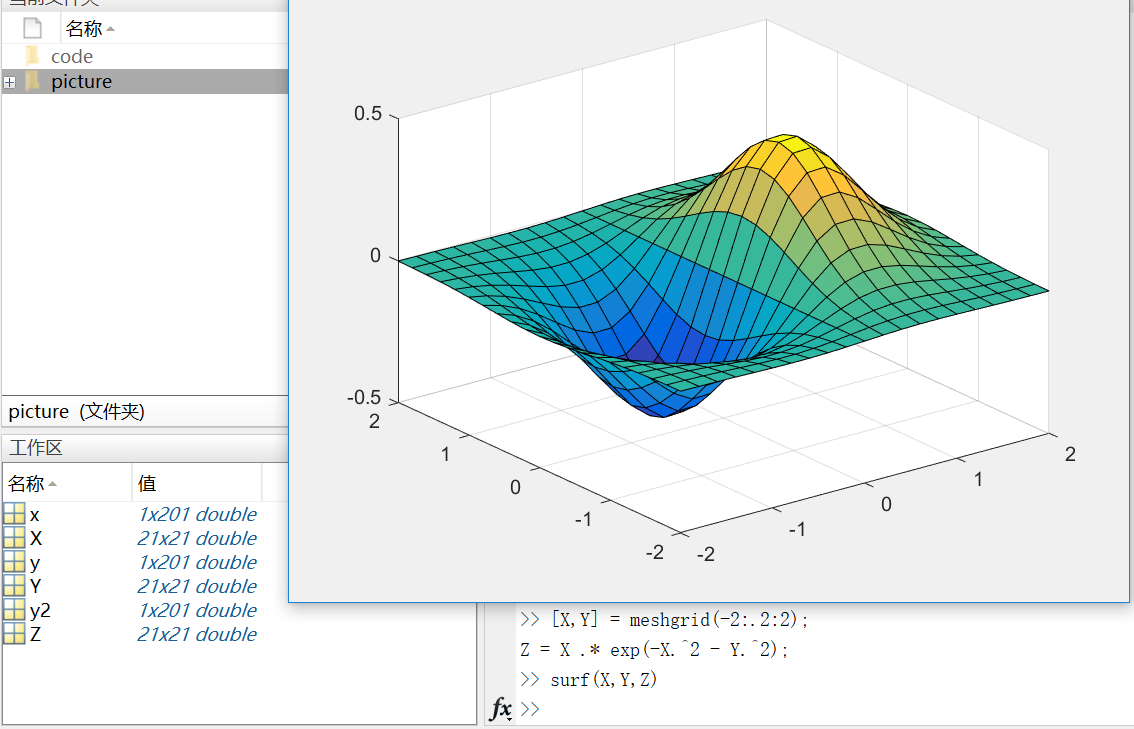这里需要注意的是，在matlab中，.2代表0.2  (-2:.2:2)表示在-2到2的区间上以0.2的长度为间隔的向量；
当变量x是由向量、数组或隔开的点组成（如x=0：0.1：1）时，则后面有x变量的方程要加点 (.)，例如：y=x.*exp(-x.*2)  但是如果x是连续的变量，如syms x，则方程写成y=x*exp(-x*2)。
Subplots  你可以用subplot函数在同一窗口的不同区域显示多个图像。  subplots函数的前两个输入参数显示当前窗口每行每列的图像数量，第三个输入参数指定活动区域。例如，在图形窗口创建四个区域来分别输出图像。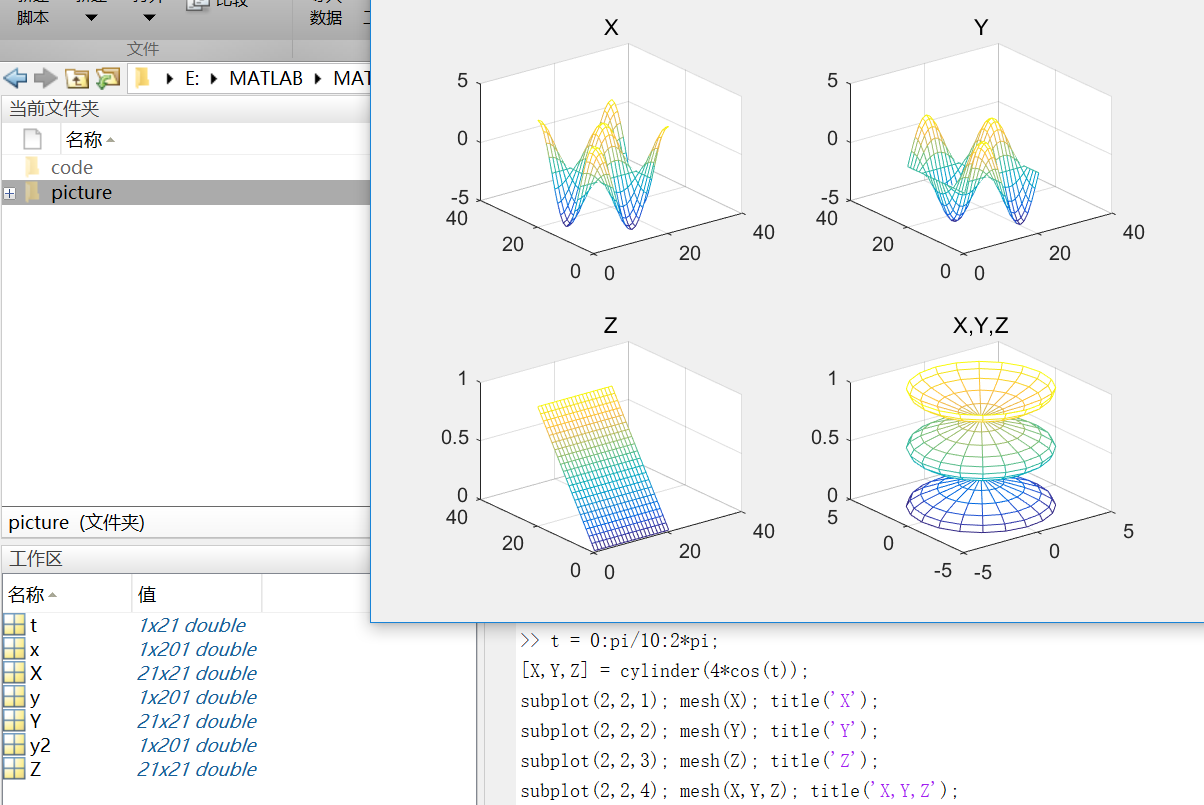mesh()生成由X，Y和Z指定的网线面，由C指定的颜色的三维网格图。
第八部分：程序和脚本
https://cn.mathworks.com/help/matlab/learn_matlab/scripts.html
示例脚本（从均匀分布中生成随机数据并计算平均值。绘制数据和平均值。）  用edit命令来创建脚本：  edit plotrand  这样就打开了一个叫plotrand.m的空文件，输入产生随机数向量的代码：
n = 50;
r = rand(n,1);
plot(r)

然后，添加代码在图中绘制水平线：
m = mean(r);
hold on
plot([0,n],[m,m])
hold off
title('Mean of Random Uniform Data')

在写代码过程中，可用（%）在代码之间添加注释。  最后保存脚本，在命令行窗口输入plotrand运行脚本。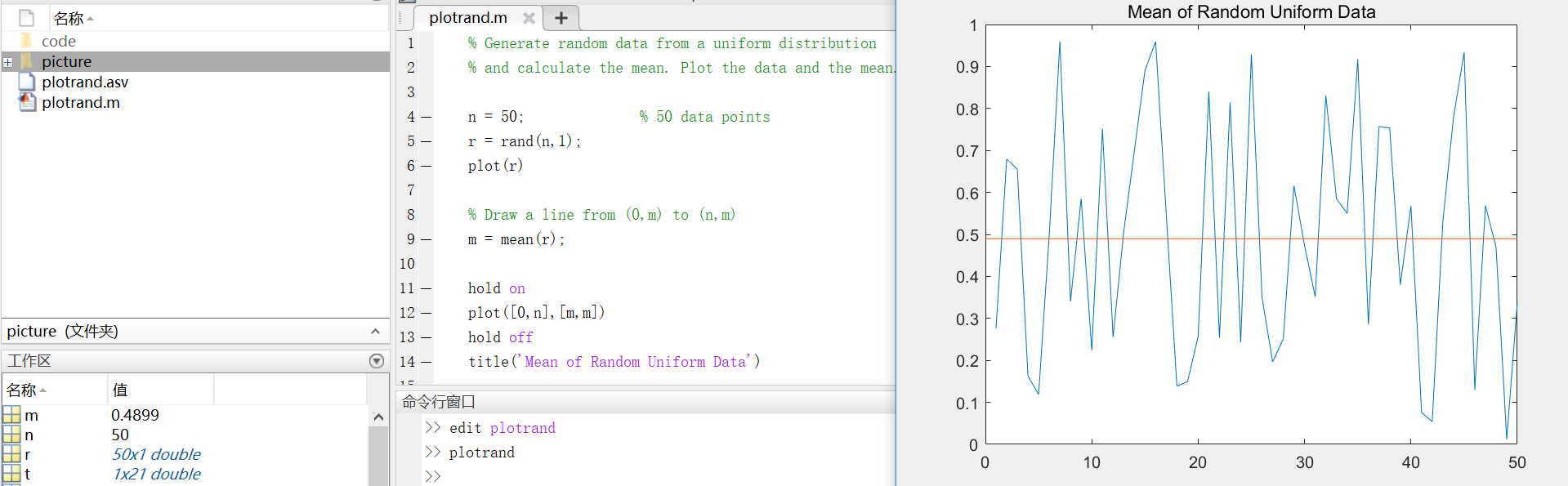循环和条件语句  在脚本中，可以循环使用部分代码，并且通过使用（for,while,if,switch等关键字）来执行这部分代码。  例如，创建一个叫calcmean.m的脚本，利用for循环计算五个随机样本的平均数和总平均数。
nsamples = 5;
npoints = 50;

for k = 1:nsamples
currentData = rand(npoints,1);
sampleMean(k) = mean(currentData);
end
overallMean = mean(sampleMean)
现在，修改for循环，以便每次迭代时都可以查看结果。在运行脚本时，会先显示中间结果，再计算总体均值。
for k = 1:nsamples
iterationString = ['Iteration #',int2str(k)];
disp(iterationString)
currentData = rand(npoints,1);
sampleMean(k) = mean(currentData)
end
overallMean = mean(sampleMean)

calcmean
在编辑器中，在calcmean.m的最后添加条件语句，使它根据不同的总体均值返回不同的信息。
if overallMean < .49
disp('Mean is less than expected')
elseif overallMean > .51
disp('Mean is greater than expected')
else
disp('Mean is within the expected range')
end
脚本位置  MATLAB中，要运行脚本，文件必须位于当前文件夹或搜索路径中的文件夹中。  默认情况下，MATLAB安装程序创建的MATLAB文件夹位于搜索路径上。如果要在另一个文件夹中存储和运行程序，需要将其添加到搜索路径中：在当前文件夹浏览器中选择文件夹，右键单击，然后选择“添加到路径”。
第九部分：帮助和文档
https://cn.mathworks.com/help/matlab/learn_matlab/help.html
使用doc命令在单独的窗口中打开函数文档。 doc mean键入左圆括号后暂停，MATLAB在命令窗口中就会显示函数提示（函数文档的语法部分）。 mean(使用帮助命令后，在命令窗口中查看函数的简单用法概括。 help mean
以上就是MATLAB的基础入门知识，欢迎指正呦~
展开全文• 这是一个不错的matlab教程，里面内容丰富 可以给初学者很好的建议...

# matlab教程matlab 订阅